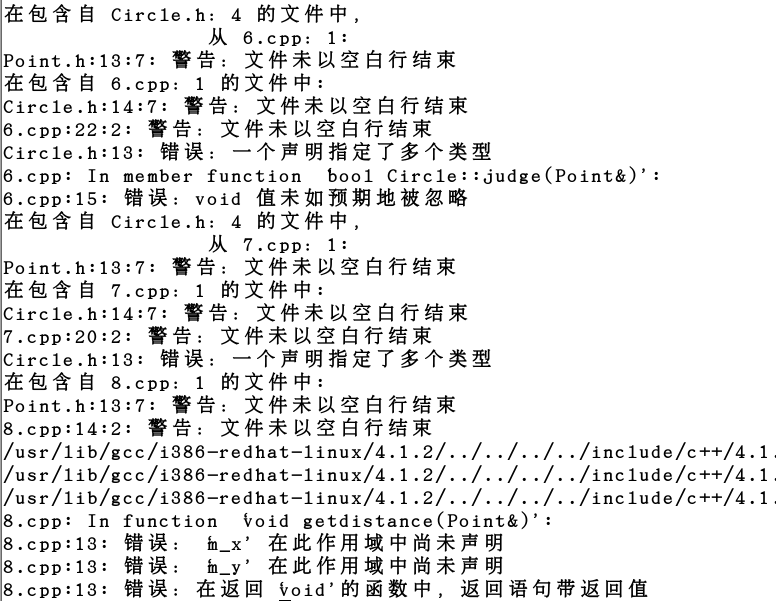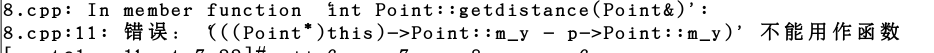• //定义一个Point类，其属性包括的坐标，提供计算 ...//判断两个是否相交并输出结果 #include&lt;iostream&gt; using namespace std; class Point { public: void setPointt(int _x1,...
//定义一个Point类，其属性包括点的坐标，提供计算
//两点之间距离的方法
//定义一个Circle类，其属性包括圆心和半径
//创建两个圆形对象，提示用户输入圆心坐标和半径，
//判断两个圆是否相交并输出结果
#include<iostream>
using namespace std;
class Point
{
public:
void setPointt(int _x1, int _y1)
{
x1 = _x1;
y1 = _y1;
}
int getX1()
{
return x1;
}
int getY1()
{
return y1;
}

private:
int x1;
int y1;
};
class Circle
{
public:
void setCircle(int _r, int _x0, int _y0)
{
r = _r;
x0 = _x0;
y0 = _y0;
}

//类做函数参数最好用引用
//在圆内返回1，不在圆内返回0
//在成员函数内可以使用c1的私有属性
int judge(Point &p)
{
int dd;
dd = (p.getX1() - x0)*(p.getX1() - x0) + (p.getY1() - y0)*(p.getY1() - y0);
if (dd < r*r)
{
return 1;
}
else
{
return 0;
}
}

private:
int r;
int x0;
int y0;

};

int main02()
{
Circle c1;
Point p1;
int tag;
c1.setCircle(2, 3, 3);
p1.setPointt(7, 7);
//点在圆内为1 不在圆内为0
tag=c1.judge(p1);
if (tag == 1)
{
cout << "在圆内" << endl;
}
else
{
cout << "在圆外" << endl;
}
system("pause");
return 0;
}

展开全文• **【题目】**判断二维空间中的，是否圆内（输出：该点在圆内、该点在圆上、该点在圆外）。 允许的误差为1e-6. **输入格式要求："%f,%f" “%f” “%f,%f” 提示信息：“请输入圆的圆心坐标：” “请输入圆的半径...
前言
判断二维空间中的点，是否在圆内（输出：该点在圆内、该点在圆上、该点在圆外）。 允许的误差为1e-6. **输入格式要求："%f,%f" “%f” “%f,%f” 提示信息：“请输入圆的圆心坐标：” “请输入圆的半径：” “请输入要判断的点的坐标(x,y)：” **输出格式要求：“该点在圆内\n” “该点不在圆内\n” “该点在圆上\n”
程序示例运行如下： 请输入圆的圆心坐标：3.5,4.5 请输入圆的半径：6 请输入要判断的点的坐标(x,y)：5.5,7.8 该点在圆内
程序源码
#include <stdio.h>
#include <math.h>
int main()
{
float a,b,r,x,y;
double m,n;
printf("请输入圆的圆心坐标：");
scanf("%f,%f",&a,&b);
printf("请输入圆的半径：");
scanf("%f",&r);
printf("请输入要判断的点的坐标(x,y)：");
scanf("%f,%f",&x,&y);
m=pow(x-a,2);
n=pow(y-b,2);
if (m+n<r*r)
printf("该点在圆内\n");
else if (m+n>r*r)
printf("该点不在圆内\n");
else if (m+n==r*r)
printf("该点在圆上\n");
return 0;
}

展开全文c语言
• 编写c++程序用来判断点在圆内（包括圆上）还是圆外，要求用坐标轴判断。    Point.h #ifndef _POINT_H #define _POINT_H class Point { private: int m_x; int m_y; public: void setxy(int x,int y);...

编写c++程序用来判断点在圆内（包括圆上）还是圆外，要求用坐标轴判断。

Point.h
#ifndef _POINT_H
#define _POINT_H
class Point
{
private:
int m_x;
int m_y;
public:
void setxy(int x,int y);
int getdistance(Point &p);
};

#endif

Circle.h
#ifndef _CIRCLE_H
#define _CIRCLE_H

#include"Point.h"
class Circle
{
private:
int m_r;
Point m_p;     //对象作为成员变量
public:
void set(int x,int y,int r);
bool judge(Point &p);
};
#endif

Point.cpp
#include"Point.h"

void Point::setxy(int x,int y)
{
m_x=x;
m_y=y;
}
int Point::getdistance(Point &p)
{
return ((this->m_x-p.m_x)*(this->m_x-p.m_x)+(this->m_y-p.m_y)*(m_y-p.m_y));
}
Circle.cpp
#include"Circle.h"
#include"Point.h"
#include<iostream>
using namespace std;

void Circle::set(int x,int y,int r)
{
m_r=r;
m_p.setxy(x,y);
}

bool Circle::judge(Point &p)
{
if(m_r*m_r>=p.getdistance(m_p))
{
return false;
}
else
return true;

}
main.cpp

#include"Circle.h"
#include<iostream>
using namespace std;

int main()
{
Circle c;
Point p;
p.setxy(1,2);
c.set(1,2,3);
if(c.judge(p))
{
cout<<"圆外"<<endl;
}
else
{
cout<<"圆内"<<endl;
}
return 0;
}
以上是正确程序。

改好之前，一堆bug（如下）。原以为要改很多，结果最后只改了两处就好了。
1.Point.h文件中class类中最后一个括号后忘记加了分号，导致Point.cpp（8.cpp）中出现许多bug。
2.Point.cpp文件中getdistance（）函数，返回值少加了一个乘号。这两处bug一共花了40分钟才搞完，没找出来之前一直以为是编译器坏了，改完之后才发现都是一些低级错误 。（下一目标：慢慢训练debug能力）
展开全文C
• 首先可以先确定一个的申明方式，后续可以通过来确定圆心。 #ifndef _POINT_H #define _POINT_H class Point {  private:  int m_x;  int m_y;  public:  void setxy(int x,int y);  int getdistance(Poi....
首先可以先确定一个点的申明方式，后续可以通过点来确定圆心。
#ifndef _POINT_H #define _POINT_H class Point {     private:          int m_x;          int m_y;     public:         void setxy(int x,int y);         int getdistance(Point &p); };
#endif

点的函数的实现
#include"Point.h"
void Point::setxy(int x,int y) {     m_x=x;     m_y=y; } int Point::getdistance(Point &p) {     return ((this->m_x-p.m_x)*(this->m_x-p.m_x)+(this->m_y-p.m_y)*(m_y-p.m_y)); }

圆的头文件
#ifndef _CIRCLE_H #define _CIRCLE_H
#include"Point.h" class Circle {     private:         int m_r;         Point m_p;     //对象作为成员变量     public:          void set(int x,int y,int r);          bool judge(Point &p);     }; #endif

圆的函数的实现
#include"Circle.h" #include"Point.h" #include<iostream> using namespace std;
void Circle::set(int x,int y,int r) {     m_r=r;     m_p.setxy(x,y); }
bool Circle::judge(Point &p) {     if(m_r*m_r>=p.getdistance(m_p))     {         return false;     }     else          return true;
}
main函数
#include"Circle.h" #include<iostream> using namespace std;
int main() {     Circle c;     Point p;     p.setxy(1,2);     c.set(1,2,3);     if(c.judge(p))     {         cout<<"圆外"<<endl;     }     else     {         cout<<"圆内"<<endl;             }     return 0; }

展开全文• #include "Stdio.h" main() { int ix,iy; printf("please input a point coordinates :");//coordinates是坐标 scanf("%d%d",&...点在圆里\n" ); els...
• 判断点是否矩形或内的方法，用与游戏开发中判断人物或技能的碰撞。
• #include #include int main() { int a,b,c; c=(a-2)*(a-2)+(b-2)*(b-2); printf("输入x，y的值:\n"); scanf("%d %d",&a,&b); if(c>1) printf("该点在\n");... printf("该点在圆内\n");
• 判断点是否在圆上(java) package zuoye; class Point{ double x; double y; //设置点的x坐标 public void setX(double x){ this.x = x; } //设置点的y坐标 public void setY(double y){ ...java
• （3）点在圆外 c++代码实现： #include <iostream> using namespace std; //判断点和圆的位置关系 //点类 class Point { public: //getset方法 void setX(int x){ m_X=x; } int getX(){ ...c++
• 判断点是否在圆内思路相似，将P(x,y)的x代入此椭圆方程（x-x1)^2/A^2+(y-y1)^2/B^2=1;得到解（2个，1个，无解）。无解，当然不椭圆内。有解，P点的y值是否[Min（解集合）,Max（解集合）]之中，若椭圆...
• 为了判断一个是否我所展示的内外，可以调用 BMapLib.GeoUtils.isPointInCircle(point,circle)//point为目标，circle为目标,调用前请先引入百度js GeoUtils.js //地图添加 var mPoint = new BMap....
• 判断点的位置关系 第一步，创建一个类，代表二维空间的一个点。二维空间确定一个点可参考平面直角坐标系中，确定了x坐标和y坐标，即可确定点的位置 package com.tyl.homework; import java.util.Scanner; /** ...java
• * @fileoverview GeoUtils类提供若干几何算法，用来帮助用户判断点与矩形、 * 圆形、多边形线、多边形面的关系,并提供计算折线长度和多边形的面积的公式。 * 主入口类是GeoUtils， * 基于Baidu Map API 1.2。 ...api 百度地图
• 20180308 java java面向对象-判断点和圆的关系判断点和圆的关系(在圆外圆周上、圆内)//练习程序//点对象class Point{ private int x;//横坐标 private int y;//纵坐标 Point(int x,int y) { this.x = x;...java课程
• 点在圆外； 3.点在圆上 设计一个C++程序，输入一个圆的坐标和半径，以及一个的位置坐标，实现相对位置的判断。如下图所示： using namespace std; #include<iostream> #include<cmath> class dot ...c++
• 平面上，如果已知△P0P1P2\triangle P_0 P_1 P_2的三个顶点坐标P0(x0,y0), P1(x1,y1), P2(x2,y2)P_0(x_0, y_0),\space P_1(x_1,y_1),\space P_2(x_2,y_2)和另一点PP的坐标(x,y)(x,y)，要判断点P是否△P0P1P2\...几何算法 向量积
• 判断一个是否指定的内，c sharpe codec#
• 描述：判断点与图形的位置关系 题目描述： 以点（point）类为基类，重新定义矩形类和类。点为直角坐标点，矩形水平放置，由左下方的顶点和长宽定义。由圆心和半径定义。派生类操作判断任一坐标点是图形内，...
• 圆外一点做圆切线的切点坐标（算法） 求圆外一点做圆切线的切点坐标（算法） 解此题的常规方法是圆的方程和切线方程联立解的两个的坐标，这种方法过于繁琐，而且代码中不易实现。由此想到用向量旋转来解此题...
• 三角形外接圆心叫外心。 /// /// Returns true if the point (p) lies inside the circumcircle made up by points (p1,p2,p3) /// /// /// NOTE: A point on the edge is inside the circumcircle...C# 计算几何
• 如题： IsPtInPoly.java ... import java.util.ArrayList;...import java.util.List;... * java判断某个是否所画范围内(多边形【isPtInPoly】/圆形【distencePC】) * @param point 检测 * @param pts
• 比如我有一个 经纬度，我想知道这个圆内还是圆外 该如何断定呢？有没有什么公式？
• 的头文件#ifndef __CRICLE_H__ #define __CRICLE_H__ #include "point.h" class Circle ... //判断点是否 当前 内 bool inCircle(Point &amp;p); private: Point _c; int ...
• 如何判断一个是不是方框（CGRect）、（Circle）、三角形（Triangle）内呢？ 1.方框 //苹果官方方法可以判断 + (BOOL)point:(CGPoint)point inSquareArea:(CGRect)rect { return CGRectContainsPoint...ios
• 计算并判断点的位置关系。 无分区版 ： #include <iostream> using namespace std; //设置 点 类 class Point { private: int p_x; int p_y; public: //设置横坐标 void setX(int x){ p_x = x; }...c++
• 设计一个圆形类（Circle），和一个类（Point），计算和圆的关系（圆上、圆外、圆内）。 例程如下： #include<iostream> #include<string> using namespace std; //一个类（Point） class ...c++...